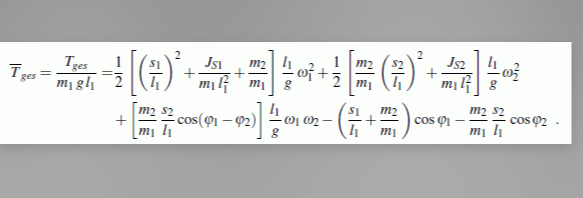# Math/Physics help with super complicated problemanyone want to help me?

4 Likes

4 Likes

3 Likes

is this a joke problem or like actual homework or something

3 Likes

nah, not a problem, i solved this alr, it was just homework, its eez

3 Likes

Is that Physics?

2 Likes

yes, but we have that in math aswell

3 Likes

Moved it from my AMA due to it does not fit to the AMA discussion

4 Likes

solve this:
x^2+x^2+x^2+x^2+x^2+x^2+x^2+x^2+x^2+x^2+x^2+x^2+x^2+x^2+x^2+x^2+x^2+x^2+x^2+x^2+x^2+x^2+x^2+x^2+x^2+x^2+x^2+x^2=42069

4 Likes

x ≈ ±39.496

2 Likes